Registration-Inscripción

March 2014

from Mid-Atlantic Geomancy Website

If we want to talk with God/dess, experience has shown that it helps to be in the right environment.

Spiritual seekers from Mayans through Christians, Native Americans, Egyptians and Hindus to the Neolithic builders of the stone rings in Britain and Ireland (and many more) found that by constructing their sacred places using certain geometrical ratios - just a small handful of them - they could more easily connect with their Maker.

Yes, it is possible to speak with our Creator anytime. However, sacred geometry makes this easier, and different ratios make different connections easier.

The ratios have to do with different spiritual activities like healing, foretelling the future, long-distance communication, levitation and, most important, heightened ability to communicate with our Maker.

These ratios help us to vibrate at the appropriate frequency to aid us in accomplishing the particular spiritual activity we have in mind.

Nearly every ancient archaeological site predating recorded history, from the Pyramids of
Egypt and Mexico, to Stonehenge and beyond, employs mysterious mathematical alignments throughout their design.

These architectural formulas, rarely used today, are considered sacred and have also been found in the way they're arranged relation to each other and, most inexplicably, in the
Monuments of Cydonia, and the Face on Mars.

When one looks at sacred enclosures globally, there is a group of five mathematical ratios that are found all over the world from Japan's pagodas to Mayan temples in the Yucatan, and from Stonehenge to the Great Pyramid.

These ratios are:

• Square Root of Two   = 1.414...

• Square Root of Three = 1.732...

• Square Root of Five   = 2.236...

• Phi = 1.618...
Phi is the Golden Section of the Greeks. It was said to be the first section in which the One became many.

• Pi   = 3.1416...
Pi is found in any circle. If the diameter is 1, the circumference is 3.1416 (C = D).

These are all irrational numbers. Pi can be taken to 1500 decimal places with no discernable pattern to it (is that Chaos?).

Let's take a closer look at each of these special numbers, and see how we can find them in the sacred geometry used by geomancers around the world.

All five of these numbers gain their meaning only when beaten against the One. They are all ratios of x:1. The One is where it begins.

Pi - 3.1416: 1 - the Circle

 Pi (3.1416 : 1) is found in any circle. In sacred geometry, the circle represents the spiritual realms.   A circle, because of that transcendental number pi, cannot be described with the same degree of accuracy as the physical square.   The circle is yin. It is a good shape to do all kinds of spiritual activities in. It is good for groups to work in circles. There are many examples of sacred spaces that are circular.The Circle: Radius (CD) = 1 Diameter (AB) = 2 Circumference = pi (3.1416) x DiameterRing of Brodgar, Mainland Orkney. Most stone rings in the British isles are not actually circular.   Dr Alexander Thom proved this with his pioneering work in the sixties.   Some of the true circles are Merry Maidens in Cornwall, Stonehenge and the Ring of Brodgar.

Square Root of Two - 1.414: 1 - the Square

 In sacred geometry, the square represents the physical world.   It can be defined totally.   If its side is one, its perimeter is exactly four, and its area is one square - exactly.   The Square is yang.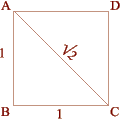The Square Side (AB) = 1 Diagonal (AC) = Square Root of Two, 1.414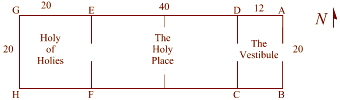The Square Side (AB) = 1 Diagonal (AC) = Square Root of Two, 1.414 The square was found was in the Holy of Holies (the back room) of Solomon's Temple (G,H,F,E).   This was where the Hebrews kept the Ark of the Covenant and other most sacred treasures. (The dimensions here are taken from the first part of the Ezekiel Chapter 41.)
 On top of Glastonbury Tor sits an impressive stone tower.   The Tor and its tower dominate the Somerset Levels.   This is a view taken from inside the tower looking upward.
Square Root of Three - 1.732: 1 - Vesica Pisces

The Vesica Pisces is created by two identical intersecting circles, the circumference of one intersecting the center of the other.

The vulva-shaped space thus created is called the Vesica Pisces.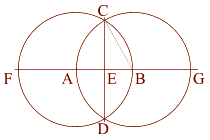The Vesica Pisces:
Two Circles share a common radius (AB).
Radius AB = 1
The intersecting circles create a Vesica Pisces.
The minor axis of this Vesica Pisces (AB) = 1,
The major axis (CD) = the square root of three, 1.732

 CB = AB = 1 ..... Therefore: a˛ + b˛ = c˛ .5˛ + x˛ = 1˛ x˛ = .75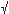2 =.75 x = .8660 = CE CE is 1/2 of the major axis CD 2 CE = CD .8660 * 2 = CD CD = 1.7320 =3

 This is the lid of the Chalice Well designed by Bligh Bond in the early part of this century.   It covers one of the most powerful Holy Wells in Britain.   The Chalice Well has numerous examples of vesicas.Gothic arch on the tower on the Glastonbury Tor. This site was a hermitage and retreat for early Christian monks
Gothic arch in Gallilee of Glastonbury Abbey. Note circular Romanesque arches behind in the Mary Chapel. The top half of the Vesica Pisces is the Gothic Arch - see Chartres Cathedral.   It is the sacred geometric shape of the Piscean Age.

Square Root of Five - 2.236 : 1 - the Double Square

 The Double Square is found in some of the best known sacred spaces in the world, from the King's Chamber in the Great Pyramid and Solomon's Temple in the Bible to the interior of Calendar II, an important underground stone chamber in Vermont, USA. The diagonal of a double square is to the shorter side as the square root of five is to one.   The square root of five = .618 + 1 + .618.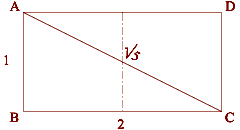The Double Square: Short Side = 1 Longer Side = 2 Diagonal = Square Root of Five, 2.236(ABCD) Double Square in Solomons Temple Solomon's temple provides numerous examples of sacred geometry.   The holy place (EFCD) is the place where good Jews who had been properly cleansed could go.   This space measures twenty cubits by forty cubits.

Another place where a double square is found is the Calendar II underground chamber site in central Vermont in the USA. It measures ten feet by twenty feet.
Calendar II, a drystone walled underground stone chamber in central Vermont, USA.The interior of the chamber is 20 feet long by 10 feet wide , or 2 to 1. The chamber is oriented towards the Winter Solstice Sunrise.

Phi - 1.618:1 - Ř - the Phi RectangleThe Golden Section, Phi, 1.618: The shorter section on the right = 3 The longer section = 5   The shorter is to the longer as the longer is to the whole 3:5 : : 5:8 In the Beginning was the One. In order to observe itself, it cut part of itself away to make 'Other'. This Golden Section is in beautiful proportion. As the subdividing continued away from the One, they continued in this phi ratio.   This can be used to go back to the One as well. It is in this sense that three is farther away from the One than two is. Have you ever noticed that it is easier mathematically to go away from One than to go towards it?   In other words, it is easier to add and multiply than it is to subtract and divide.

3:5 : : 5:8. This ratio indicates that it is part of this series: 1 . 2 . 3 . 5 . 8 . 13 . 21 . 34 . 55 . 89, and so on.

This is called the Fibonacci Series. Start anywhere in the series, add the number below, and you get the next number (for example, 21 + 13 = 34).

As one ascends up the series, any number in the series, when divided into the next one up, gets closer and closer to (but never hits exactly) 1.618, phi, the Golden Section.

On a line create square
(ABCD) where AB = 1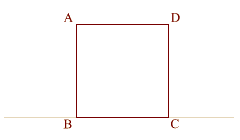Divide lines (AD) and (BC) in half
at (F) and (E). (BC) = 1, (EC) = .5

Double square (ECDF) is thus
created with a diameter of (ED).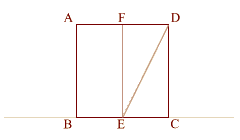Using (ED) as a radius
swing arc from (D) downwards to 0
intersect the initial base line at (G).

Extend line (AFD), and create a
perpendicular to line (BECG) at (G)
so that it intersects line (AFD) at (H),
thus creating phi rectangle (ABGH).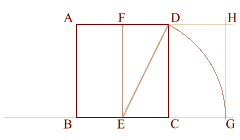The formula that shows this is:Phi = ( 1 + square root of 5 ) divided by 2

(BE) = 1/2
(ED) = 5/2
.5 + 1.118 = 1.618

Extend arc (DG) through (A) to (I).
Note the clear relationship between
phi and the square root of five.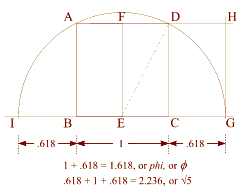Solomon's Temple also contains phi.   The Vestibule (DCBA) measures twelve cubits by twenty cubits. 12 to 20 can be reduced to 6 to 10 and further to 3 to 5.   Three and five are two numbers in the fibonacci series. 3/5 = 1.6, a close approximation to 1.618, or phi.
 Calendar: I was measured very carefully by the NEARA/ASD Earth Mysteries Group in the early 80's.   Three measurements of the length were taken and averaged.   The same was done with the width. Upon dividing the length by the width, the resultant ratio was 1.619 to 1. Phi (Ř) = 1.618 to 1.
The Parthenon is the Queen of Greek Temples, and personifies their interest in Sacred Geometry. If the height of the Parthenon is 1, its width is phi (Ř) 1.618, and its length is root of 5= 2.236. And 1.618 + .618 = 2.236. These are the 5 sacred geometrical ratios - Pi, (2),(3),(5) and Phi. They are found in sacred spaces all over the world.   Remember, sacred geometry is basically simple. You must do it with your hands, if you want to really know sacred geometry.

Squaring the Circle - The Great Pyramid

 The square represents the physical. The circle represents the spiritual.   All sacred geometers have attempted the impossible: to square the circle (create a square who's perimeter is equal to the circumference of a circle.)   Here is the first of two valiant attempts: This squaring of the circle works with a right triangle that represents the apothem (ZY) - (a line drawn from the base of the center of one of the sides to top of the pyramid), down to the center of the base (ZE), and out to the point where the apothem touches the Earth (EY).
The Great Pyramid of Egypt (Sphinx in foreground)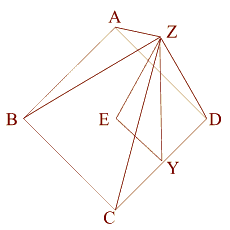Now let's look at this in 2D, from directly above. For the purpose of this exercise, the side (AB) of the base equals 2.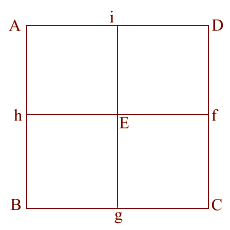(ABCD) is the base of the Great Pyramid. This is lettered similarly to the wire frame version (above). For the purpose of this exercise, the side (AB) of the base equals 2.Construct square (i JKD), thus creating double square (JKE f).Create diagonal (EK) which intersects (i D) at (l). iD = 1, therefore the diameter of the circle is also 1. (EK) = (5) = .618 + 1 + .618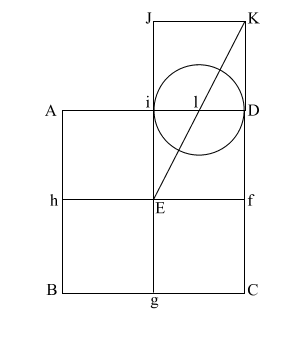Put the point of your compass at (E) and extend it along the diagonal (EK) to point (m) where the circle intersects (EK), and draw the arc downward to intersect (KD f C) at (n). If (EK) = (5), and (l m/l D) and l i = .5, the diameter of this circle is 1.   This makes (E m) = .618 + 1, or 1.618. (E m) is the apothem.Draw (E n) which intersects (A i l D ) at (o). Put compass point at (f) and extend it to (n). Again put your point at (E) and draw the circle which happens to have the radius (E o). (f n) is the height of the Great Pyramid. This circle comes remarkably close to having the same circumference as the perimeter of the base (ABCD).

Let's go back to the original right triangle (EYZ)
(EY) = .5

(YZ) = phi

(EZ) = (phi)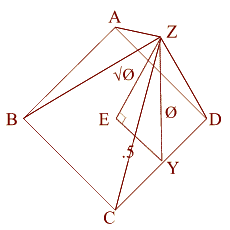EY = .5, The apothem is phi/1.618. This makes the 51 degree + degree angle.

Using a˛ + b˛ = c˛, this makes the height the square root of phi.

Squaring the Circle - The Earth & the Moon

 Create a square (ABCD) with (AB) = 11 Create diagonals (AC) and (BD) crossing at center point (E) Construct a circle which is tangent to square (ABCD) at f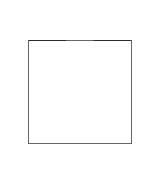Construct two 3 . 4 . 5 right triangles, with the 4 . 5 angles at (A) and (D). Connect the 5 . 3 angles creating square (abcd) with side (ab) = 3 {4 + 3 + 4 = 11, or side (AD) of square (ABCD)} Create diagonals (ac) and (bd) centering at (e) Create a circle that is tangent to square (abcd) at four places.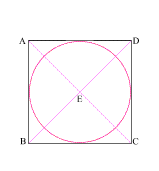Draw line (Ee) which intersects side (AD) at (F) (EF) = the radius of the larger circle and (eF) = the radius of the smaller circle The larger circle thus created is to the smaller circle as the moon is to the Earth! With your compass point at (E), create a circle with radius (Ee) This creates a circle whose circumference is equal to the perimeter of square (ABCD)!The Math

 1 (AB) = 11 (EF) = 1/2 of (AB) = 5.5 (ab) = 3 (eF) = 1.5 Therefore   5.5 + 1.5 = 7 The circumference of a circle is equal to two times the radius (the diameter) times pi (3.1416). C= 14 x 3.1416 C= 43.9824 2 In Square (ABCD), (AB) = 11 The perimeter of a square is four times one side. 11 x 4 = 44 According to the Cambridge Encyclopedia, the equator radius of the Earth is 3963 miles. The equator radius of the Moon is 1080. The claim is that the smaller circle (in square abcd) is to the larger circle (in square ABCD) as the Moon is to the Earth.
 3 (EF) = 5.5 (F e) = 1.5 5.5 : 1.5 :: 3963 : 1080 5.5 / 1.5 = 3.66666 3963 / 1080 = 3.6694 - (if it had been 3960, it would have been exact!)

Sacred Geometry

Geomancers are interested in sacred geometry because this is the study of the way that spirit integrates into matter - by echoing and amplifying the geometry of nature and planetary movements, we help to align the resonance of body/mind/spirit with the harmonic frequencies of the above and the below.

Geomancers are interested in sacred geometry because it has been found that certain spaces, with particular ratios, enable the participant to resonate or vibrate at the appropriate rate that maximizes the possibility of connection to the One.

A violin isn't built out of a cigar box! It is built with the proper wood with the proper shape and ratios, so that it resonates correctly for the notes/frequencies it is expected to produce.

These same principles are applied to sacred spaces to maximize the possibility that whatever is being done there on spiritual levels will succeed.

Definitions

Two Dimensions

I've been a student of sacred geometry for over twenty-five years.

While there has been recent interest in three-dimensional sacred geometry based on the Platonic Solids and in sacred sites themselves, most sacred geometrical documents I've read talk in only two dimensions - height and width.

Obviously there is a fourth dimension and others beyond it that are much more complex and sophisticated. But why does the record left to us from geomancers of the past come primarily in two dimensions?

Two is closer to the One than three is. It's less complex. I think one of the biggest mistakes Western geomancers have made was to take something that is very simple and make it much more complex.

The Chartres Labyrinth strikes me as being an example of this. This stuff is simple. If you really know (that is, know both rationally and intuitively) a handful of irrational ratios - pi (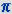), phi (Ř) and the square roots of two, three and five, you've basically got it all.

Three-dimensional sacred geometry just builds on this basic handful.

Numbers

One aspect of Sacred Geometry is that it works with irrational numbers.

To go to the spiritual, one must go beyond the rational, and it appears that some of these ratios and numbers can lead us there. By being inside a sacred space that has been constructed using one of a handful of these sacred geometrical ratios, the resonance that has been set up can enhance the possibility of your making the spiritual connection you want to make.

So, what are these irrational numbers? Let's begin with the rational.

Rational Numbers

A rational number is a number which can be expressed as the ratio of two integers (whole numbers), such as 1/3 or 37/22. All numbers which, when represented in decimal notation, either stop after a finite number of digits or fall into a repeating pattern, are rational numbers.

Irrational Numbers

An irrational number is one that cannot be represented as a ratio of any two whole-number integers, and consequently it does not fall into a repeating pattern of any sort when written in decimal notation.

All of the Sacred Geometry ratios we will be working with, the square roots of two (1.414), three (1.732) and five (2.238), phi (1.618) and pi (3.1416), are all irrational numbers.

Transcendental Numbers

There are certain kinds of irrational numbers that are called transcendental numbers.

Just like irrational numbers, they are defined by what they are not (they aren't rational numbers), yet transcendental numbers are so identified because they are not another sort of number, known as an algebraic number.

Any number which is a solution to a polynomial equation is an algebraic number. A polynomial equation is a sum of one or more terms involving the same variable raised to various powers, for example:

7 (x5) + 5 (x3) + x = 137

Any X for which any such equation is true is an algebraic number. Because the square root of two is a solution to the polynomial equation,

x2 = 2

it is an algebraic number.

A transcendental number requires an infinite number of terms to be defined exactly. That's one way of thinking of God/dess.

There are special equations to derive transcendental numbers where the terms get smaller and smaller as you go along, so you can keep adding them together to reach any level of accuracy you need, but the true number cannot be reached exactly.

That is the beauty of transcendental numbers!

Pi (= 3.1416...) is such a transcendental number. It is the only one we will be using here with Sacred Geometry. One infinite equation which relates to the value of pi () is this:

Pi / 4 = 1 - (1/3) + (1/5) - (1/7) + (1/9) - (1/11) + (1/13) - (1/15) + ...and so on into infinity.